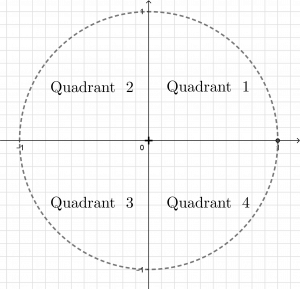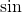# The Unit Circle

Notes/Exercise: Grade 11 Trigonometry Unit circle

When solving right angled triangles with SOH CAH TOA, we considered values of sine, cosine and tangent between zero and 90 degrees only, as follows:

However, values of sine, cosine and tangent are defined for angles between negative infinity and positive infinity.

The unit circle shown on the applet below allows us to explore trig values between zero and 360 degrees.

Notice that some trig values are positive and some are negative. We can now define the values of cosine and sine to be thevalues of a point on the circumference of the unit circle.

In words:

Let P be a point on the circumference of a circle with radius one unit and center at the origin. Draw a radius from the center to the point P. Letbe the angle between the positiveaxis and the radius, measured counter-clockwise. The coordinates of P are.

Remembering thatgives us all three ratios. This leads to a simpler diagram for the values of sine and cosine:

# Positive and Negative values of Sine, Cosine, Tangent

Values of cosine are defined as thecoordinates of the point P. These are positive on the right hand side of the diagram.

Values of sine are defined as thecoordinate of the point P.coordinates are positive in the upper half of the diagram.

Values of tangent are defined as. These are positive on one diagonal and negative on the other.

We refer to the quadrants of the Cartesian Plane as follows:The following diagram is a helpful for remembering which trig values are positive where.A simpler version of the diagram is often referred to as the CAST diagram:# Reference Angle

A reference angle is an angle in the first quadrant. In the following applet a point in the first quadrant is reflected to make a total of four points. With information from one of these points, we can calculate the sine, cosine and tangent of the other three.

Calculate the angle for each point, and compare the,coordinates given on the diagram withandvalues given for the angle on your calculator.